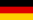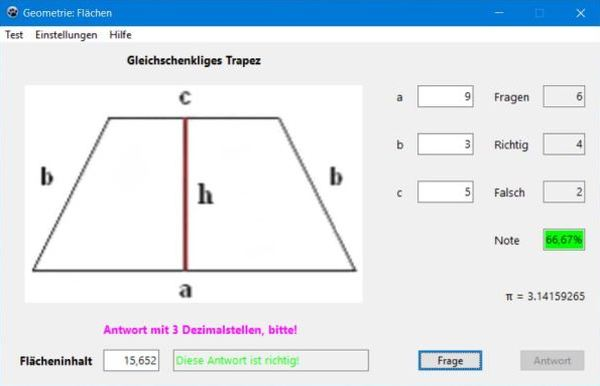# Computing: Free Pascal Programming

 Programming Home Home Contact

## Maths trainer: Surface's area and circumference (Geometry1).Description: Generation of exercises concerning the calculation of the area and the circumference of geometrical surfaces. You may choose what to calculate (Flächeninhalt = area, Flächenumfang = circumference) and also what the exercises generated are about, in particular if they should include examples that need the usage of the Pythagorean Theorem to do the calculation, as for example on the screenshot below, where h has to be calculated from b. Help text, including the formulas (in German, as the application itself) included.

Free Pascal features: Basic controls: menus, images, edit fields, (push) buttons; hiding not used controls.

Screenshot: